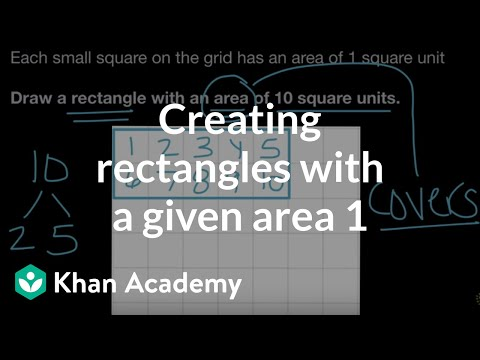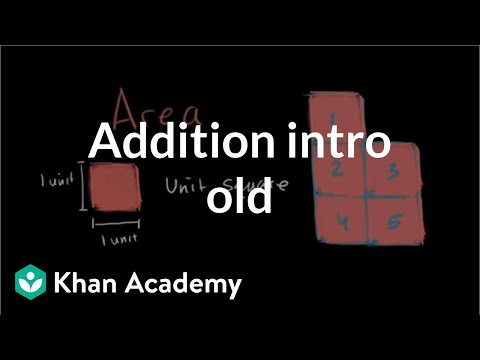Video

# Creating rectangles with a given area 2 (Full video)

Description: Lindsay creates a rectangle that has the same area (but different side lengths) than a given rectangle. Area is the amount of space a shape covers, so how much space, or how many square units does this shape cover, does our rectangle cover? So, we want to draw another rectangle that also covers eight square units. Let's create a rectangle here, two rows of four, and we can just spread this out a little bit so it covers the whole square units.

### Other videos you might be interested in### Creating rectangles with a given area 1 (Full video)